## Selecting a Monochromatic Energy

### Introduction

There are several CIAO tools that handle energy-dependent calibration issues by evaluating the instrument response at a monochromatic energy input by the user. The intent is that this energy should be a nominal value which is representative of the actual range of photon energies in the subset of data being analyzed. The hope is that the final results will depend only weakly on the precise choice of this nominal energy. Since Chandra's sensitivity spans two decades of energies (0.1 to 10 keV) with sharp, non-linearities in the response, poor selection of a single monochromatic energy can lead to significant systematic errors in the results. This why topic will discuss:

• How the monochromatic energies are typically used.
• How to compute a monochromatic energy under different constraints.
• The systematic errors that arise from the choice of a monochromatic energy.

### Monochromatic Energy in srcflux

The srcflux tool uses many of the individual tools and scripts that take a monochromatic energy as input. As such, it is a good case study to use to understand how the choice of monochromatic energy affects things.

srcflux computes the net counts, photon flux, model-independent flux, absorbed and unabsorbed model-dependent fluxes. The monochromatic energy is specified via the bands parameter. The Chandra Source Catalog (CSC) energy bands are available by name and correspond to an energy range and a nominal 'central' monochromatic energy. The ranges and monochromatic energies are shown in Figure 1..

### Figure 1. Chandra Source Catalog Energy Bands.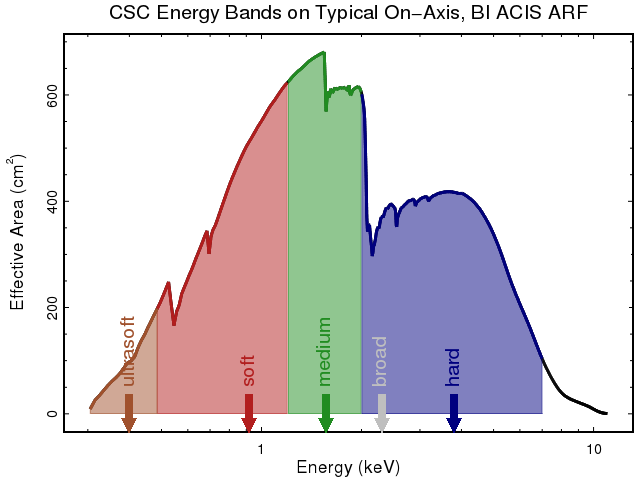### Figure 1. Chandra Source Catalog Energy Bands.

The Chandra Source Catalog (CSC) energy bands for ACIS are shown on a typical ACIS-S3, nearly on-axis, ARF. The ACIS Broad band covers the soft, medium, and hard bands combined. The arrows identify the monochromatic energy used with each band. The ultrasoft band goes from 0.2 to 0.5 keV with a monochromatic energy of 0.4; soft goes from 0.5 to 1.2 @ 0.92 keV, medium goes from 1.2 to 2.0 @ 1.56 keV, hard goes from 2.0 to 7.0 @ 3.8 keV, and the broad band goes from 0.5 to 7.0 with a monochromatic energy of 2.3 keV.

#### How does srcflux use the monochromatic energy?

• With psfmethod=quick or psfmethod=arfcorr the monochromatic energy is used to adjust the net counts for the fraction of the PSF that is included in source and background apertures. All the other fluxes are derived from the net_flux or its confidence interval limits.
• The photon flux, net_photflux_aper (photon/cm2/s), values stored in the output .flux file are computed using a monochromatic exposure map.

### How to Compute the Monochromatic Energy

There are different techniques that can be used to compute a sensible monochromatic energy based on data being analyzed.

In examples below, the goal is to pick a monochromatic energy for a source in the 2.0 to 10.0 keV range.

#### Case 0: Visual estimation

Experienced users may simply estimate the monochromatic energy based on a visual inspection of the effective area in the desired energy band. Generally this will be a value located near the maximum in the energy band and careful to avoid any absorption edges.

```unix% pset srcflux band="2.0:10.0:4.0"
```

#### Case 1: Unknown spectrum, large number of events

If the spectrum of the source is entirely unknown, but the source has a large number of counts then the mean energy of the events can be used. Here dmstat is used with an energy and spatial region file to determine the mean energy of the events in the event file. Note: units in the event file are eV, srcflux requires units in keV.

```unix% dmstat "acis_evt2.fits[sky=region(ds9.reg),energy=2000:10000][cols energy]"
energy[eV]
min:    2001.9193115          @:    36
max:    6817.7631836          @:    37
mean:    3404.581305
sigma:    1136.2303411
sum:    200870.297
good:    59
null:    0

...
unix% pset srcflux band="2.0:10.0:3.4"
...
```

With only 59 counts (the good value above) and a large standard deviation (sigma) this may not be enough events to get a good estimate of the true mean event energy.

#### Case 2: Unknown spectrum, small number of events

In this case where the spectrum is unknown and there are few counts, users will likely need to resort to using the effective area weighted mean energy. This requires the user create the ARF file by running specextract or by running srcflux once just to get the ARF and then running again once the monochromatic energy is determined using the commands shown here:

```unix% dmtcalc out.arf arf_weights expression="mid_energy=(energ_lo+energ_hi)/2.0;weights=(mid_energy*specresp)" clob+
unix% dmstat "arf_weights[mid_energy=2.0:10.0][cols weights,specresp]" verbose=0
unix% pget dmstat out_sum
750684.49471,172414.3308
unix% echo  750684.49471/172414.3308 | bc -l
4.35395649089512923481
...
unix% pset srcflux band="2.0:10.0:4.4"
...
```

#### Case 3: Known spectrum

The final option is for users that have a reasonable guess at the source spectrum. Users should already have extracted the spectrum via specextract or by following the appropriate step-by-step threads With the known model and response file the mean energy an be computed using Sherpa.

```# Load the spectrum into sherpa

# Setup the known source model and parameters
sherpa> set_source(xspowerlaw.pow1*xsphabs.abs1)
sherpa> pow1.PhoIndex=1.7
sherpa> abs1=0.03

# Set the energy range
sherpa> notice(2.0, 10.0)

# Get the model flux which include the ARF
sherpa> flux_data = get_model_plot()

# Compute the flux weighted mean energy
sherpa> egrid = (flux_data.xhi+flux_data.xlo)/2.0
sherpa> flux = flux_data.y
sherpa> mean_e = np.sum(flux*egrid)/np.sum(flux)
sherpa> print(mean_e)
3.62574319232
...
unix% pset srcflux band="2.0:10.0:3.6"
...
```

Some key srcflux results are shown below. All parameters were left at their defaults with the exceptions: band=2:10:\${energy} and psfmethod=quick. The 90% confidence intervals are shown for a source with 58 net counts in the 2:10 keV band.

Case Energy (keV) PSF Fraction Net Count Rate (counts/sec) Net Photon Flux (photon/cm2/sec)
Case 0 4.0 0.820 0.00196 (0.00154,0.00238) 4.72E-06 (3.70E-06,5.74E-06)
Case 1 3.4 0.835 0.00192 (0.00151,0.00234) 4.64E-06 (3.64E-06,5.65E-06)
Case 2 4.4 0.811 0.00198 (0.00155,0.00241) 4.99E-06 (3.92E-06,6.08E-06)
Case 3 3.6 0.829 0.00193 (0.00152,0.00235) 4.63E-06 (3.63E-06,5.64E-06)

Since the detector response does not change rapidly in the 2 to 10keV range the results are all consistent within the statistical errors. However, for different energy bands the choice of monochromatic energy can introduce large systematic offsets. These are discussed in the next section.

### Systematic Errors

The choice of a single monochromatic energy to represent an energy band will almost always introduce sources of systematic error. The magnitude and direction of this error depends on many factors such as the width of the energy band and how rapidly the response of the detector varies within that band.

Two sources of systematic errors were identified in the srcflux use case: the PSF fraction (all fluxes) and Effective areas (photon fluxes). Both of these will be discussed below.

#### PSF Fraction Uncertainty

When srcflux is run with psfmethod=quick or psfmethod=arfcorr it uses the energy band's monochromatic energy to determine the PSF correction for the source and background regions. While the PSF size (and therefore fraction within a fixed region) does change significantly with energy, the change is relatively slow. Figure 2 shows the PSF fraction for a 1.5" (~3 pixel) circular region at 0.58' off-axis vs. energy. The PSF fraction drops from 98% at 0.2 keV to 84% at 10 keV. Since the change is gradual, a small change in the assumed monochromatic energy will result in only a small change in PSF fraction (thus a small systematic uncertainty).

### Figure 2. PSF Fraction vs Energy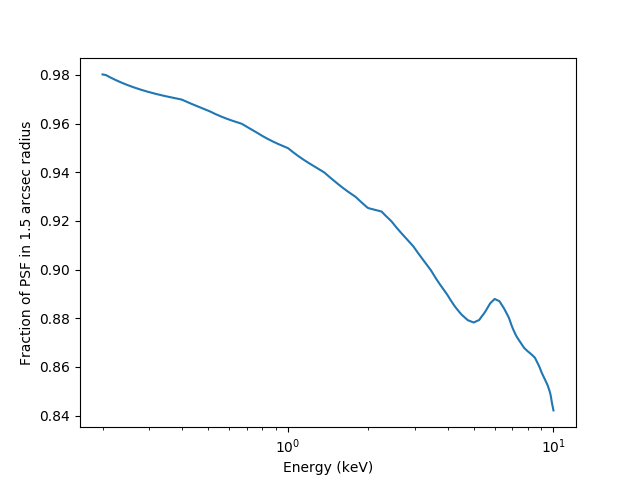### Figure 2. PSF Fraction vs Energy

The fraction of the PSF enclosed by a 1.5 arcsec circular region at 0.58 arcmin off-axis (nearly on-axis), as a function of energy. While there is a 14% decline from 0.2 to 10 keV, the slope of the decline is low. Therefore small changes in the monochromatic energy will yield only small changes in the PSF fraction and thus the fluxes. Note that even the "bump" between 5 and 7 keV is only at the 1% level.

The slope of this curve does depend on the source's location within the field of view, its off-axis angle, and the size of the region. Generally, however, it should be consistent with Figure 2. Users can generate a similar curve for their source using matplotlib and the Python PSF module as shown here

```unix% python

>>> from psf import *
>>> import os
>>> import numpy as np
>>> energies = np.arange(0.2, 10, 0.001)  #keV
>>> theta = 0.58                           # arcmin
>>> phi = 45.0                            # degrees
>>> radius = 1.5                           # arcsec
>>> psf_fraction = [ psfFrac( p, e, theta, phi, radius) for e in energies]
>>>
>>> import matplotlib.pylab as plt
>>> plt.plot( energies, psf_fraction)
>>> plt.xlabel("Energy (keV)")
>>> plt.ylabel("Fraction of PSF in 10arcsec radius")
```

With srcflux the PSF fraction is generally just a scale factor that is applied to the net_counts, its errors, and all the fluxes that are derived from them. So if there is a 1% uncertainty in the PSF fraction that results in a essentially a 1% uncertainty in the fluxes.

#### Effective Area

srcflux uses the effective area at the specified monochromatic energy to compute the net photon fluxes, photon/cm2/s, by way of the instrument map. As shown above in Figure 1, a typical ACIS effective area has several absorption features : the largest are the Iridium M-edges at ~ 2keV, the Aluminum edge at ~1.49 keV, and the Oxygen edge at ~0.536 keV. With such sharp spectral features, a small change in the assumed monochromatic energy can result in a large change in the effective area and therefore a large change in the net photon flux.

The magnitude of this systematic error can be seen by using the Known Spectrum approach described above. In Figure 3 the parameters of an absorbed powerlaw are varied and the monochromatic energy is computed following the provided recipe. srcflux is then run to compute the fluxes in the CSC broad band, 0.5 to 7.0 keV, for a source that contains ~300 net counts with the ARF shown in Figure 1.

### Figure 3. Monochromatic energy assuming an absorbed powerlaw in the broad band.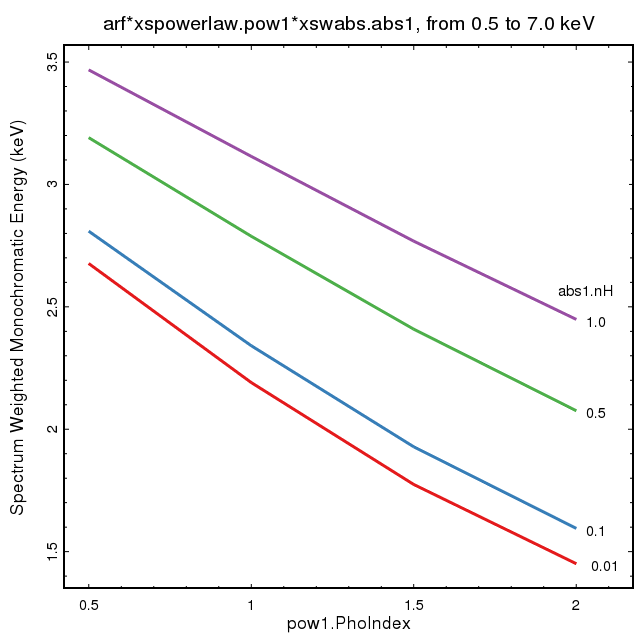### Figure 3. Monochromatic energy assuming an absorbed powerlaw in the broad band.

The parameters for an absorbed powerlaw model are varied to determine the monochromatic energy (Y-axis) using the above snipet of code. The powerlaw index is plotted on the X-axis, with individual curves shown for different values of nH.

The data in Figure 4, use the monochromatic energies in Figure 3 with srcflux to compute the Net Photon Flux (net_photflux_aper).

### Figure 4. Broad band photon flux, for monochromatic energies assuming an absorbed powerlaw.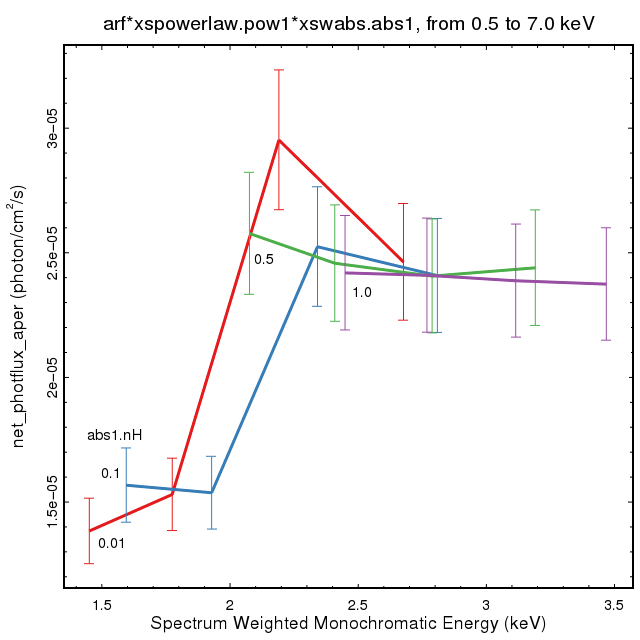### Figure 4. Broad band photon flux, for monochromatic energies assuming an absorbed powerlaw.

The monochromatic energies from the model and parameters shown in Figure 3 are used with the srcflux net_photflux_aper values plotted.

Model parameters that yield a monochromatic energy less than 2.0 keV produce photon fluxes 40% lower than models that produce monochromatic energies above 2keV. This systematic error is much larger than the statistical confidence limits for this 300 net-counts source.

This then shows how a small change in the assumed monochromatic energy can lead to large systematic offsets in the photon flux. The magnitude of the error is directly related to the magnitude of the change in the effective area within the selected energy band.

Choosing a different energy band where the effective area changes gradually will result in less of a systematic uncertainty. Figure 5 and Figure 6 show the same model and parameters but for the 0.5 to 1.2 keV energy band (CSC soft band).

### Figure 5. Monochromatic energy assuming an absorbed powerlaw in the soft band.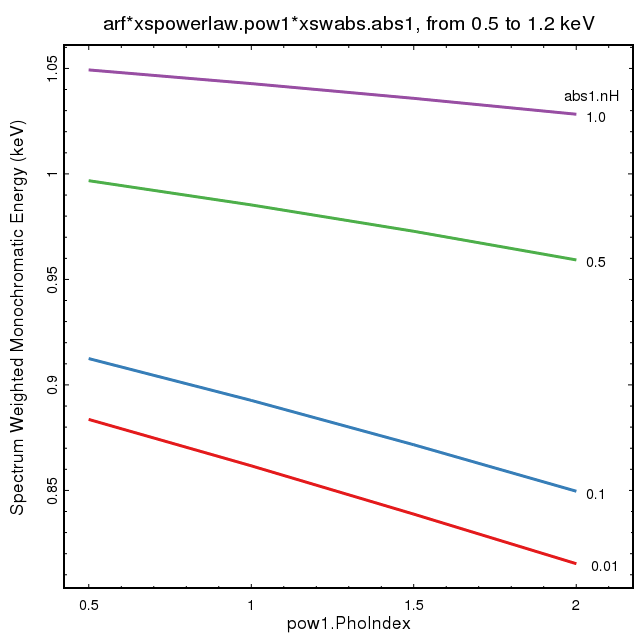### Figure 5. Monochromatic energy assuming an absorbed powerlaw in the soft band.

Same as Figure 3 but for the CSC soft band: 0.5 to 1.2 keV.

### Figure 6. Soft band photon flux, assuming an absorbed powerlaw.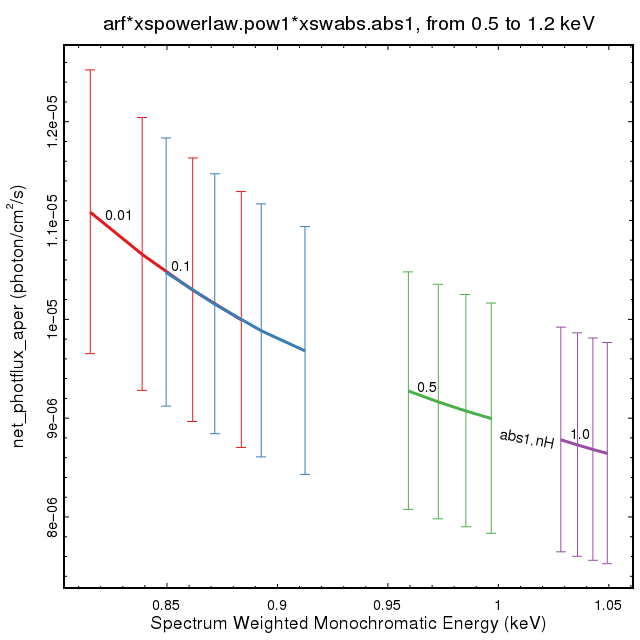### Figure 6. Soft band photon flux, assuming an absorbed powerlaw.

Same as Figure 4 for the soft band.

Since the effective area changes more gradually in this energy band, changes in the assumed monochromatic energy produce smaller systematic offsets. The results are within the 90% confidence limits for the 160 counts in this energy band.

The choice of spectral model itself only affects the photon fluxes in how it affects the change in monochromatic energy. The same analysis is repeated with an absorbed blackbody with varying temperatures. The results are shown in Figure 7 and Figure 8.

### Figure 7. Monochromatic energy and photoflux assuming an absorbed blackbody in the broad band.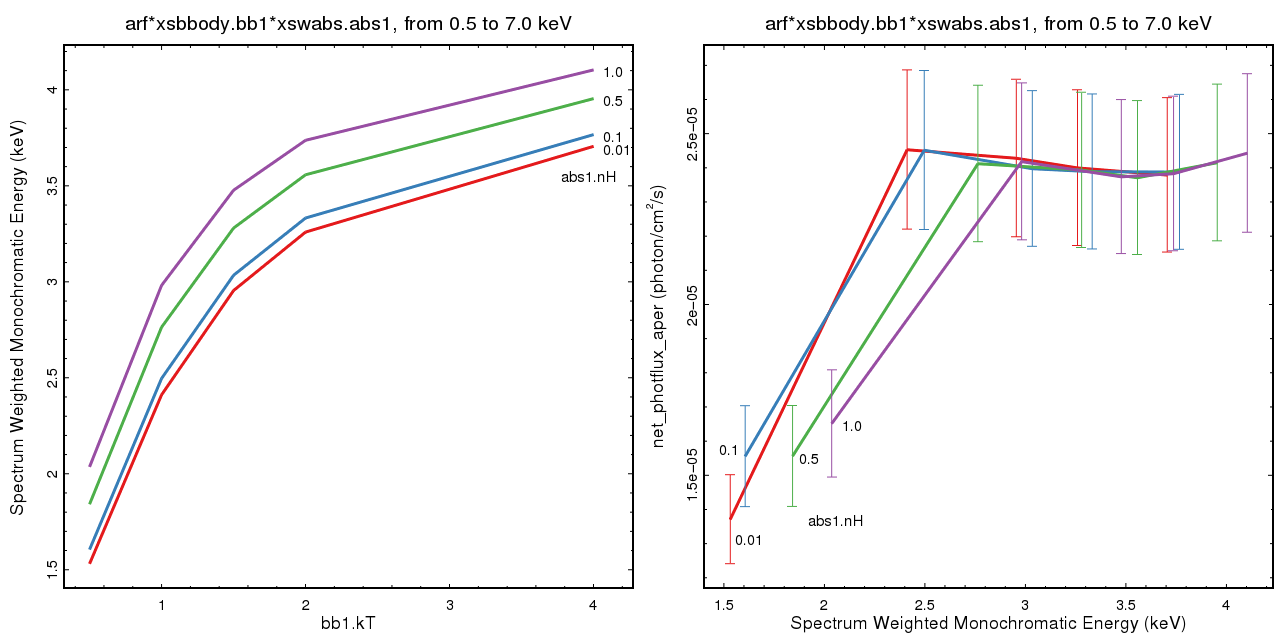### Figure 7. Monochromatic energy and photoflux assuming an absorbed blackbody in the broad band.

Same as Figure 3 and Figure 4 but for an absorbed blackbody with different temperatures.

(Left) shows monochromatic energy vs. model parmaters computed over the broad band. (Right) shows the photon flux which is similar to the powerlaw model.

### Figure 8. Monochromatic energy and photoflux assuming an absorbed blackbody in the soft band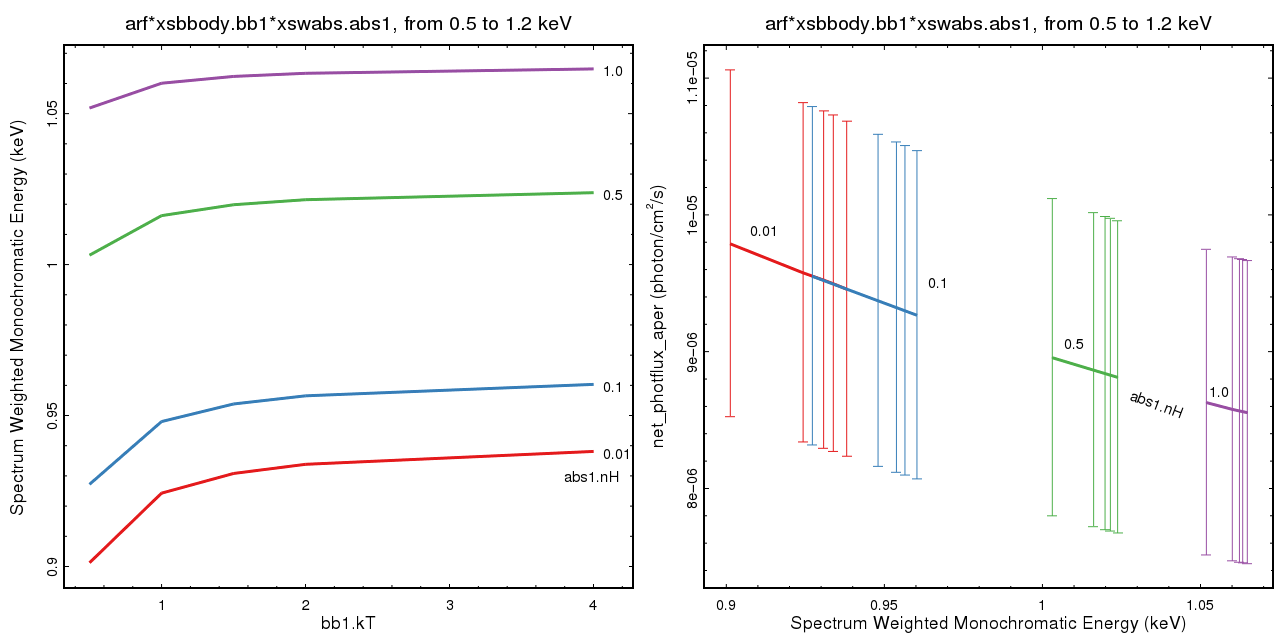### Figure 8. Monochromatic energy and photoflux assuming an absorbed blackbody in the soft band

Same as Figure 5 and Figure 6 but for an absorbed blackbody with different temperatures.

(Left) shows the monochromatic energy vs model parameters computed over the soft energy band. (Right) shows the photon flux vs. energy.

### Summary

Several CIAO tools require a characteristic, monochromatic energy as an input parameter.

This page has shown several methods to compute this value based on the data being analyzed -- the brightness of the source and any a priori knowledge about the spectrum of the source.

The choice of the monochromatic energy can lead to systematic uncertainties in the analysis. Two of these errors have been examined in the context of the srcflux tool. Changes in the monochromatic energy may affect the PSF fraction calculations which affects all the flux measurements; however, since the PSF fraction changes slowly with energy, this is generally not a significant source of error. A larger systematic error may be seen when working with the photon flux values that use the effective area. With various absorption edges contributing to the effective area, a small change in monochromatic energy can lead to large systematic changes in the photon fluxes. This systematic uncertainty in the photon fluxes can be minimized by selecting energy bands where the effective area varies slowly.

Users concerned about such systematic errors either in the PSF fraction or in the photon fluxes are encouraged to repeat their analysis with different monochromatic energies to determine the effect it has on their specific work.